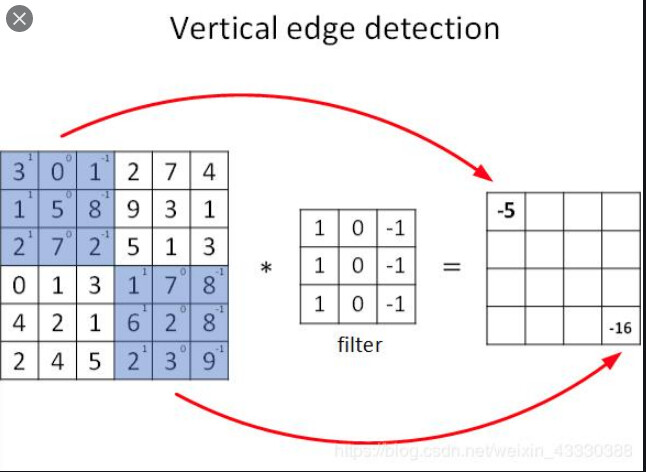# Are convolutions actually multiplying over nxn grids?

Asking - as I think I’ve mis-understood something; so likely a few inaccuracies in my below description.

A common image for explaining a CNN filter shows it being applied over a sub grid in an image such asAnd convolutions are defined with a width and height.

However; since images are flattered before they are processed; and fully-convolutional networks can work on any image size & aspect ratio, and we don’t tell models the original width of an image - how would a 2x2 filter be applied to 2 pixels in the first row and 2 pixels from the second row of the original image?

For example; if the input image is 240x480; then the first grid of pixels would be 0,1,240,241 in the flat array, whilst if an image was 640x480 the offsets would be 0,1,640,641.

I don’t agree with the assumption that “images are flattened before they are processed”. Why would you assume that? And if that is what PyTorch is doing in the background of `nn.Conv2d`, then it’s doing that on its own for optimization reasons, but it’s fully aware of the input tensor shape which includes the tensor height and width.

Right; that would make sense. I think I was confusing myself from earlier chapters in the fastai course which are not using convolutional nodes and do have a “flattern” image step; so I must look into the details of `mnist = DataBlock((ImageBlock(cls=PILImageBW), CategoryBlock)` to understand it better.

Images are flattened before they are processed because computer memory is linear.A convolution is a bunch of dot products. A matrix multiplication is also a bunch of dot products. So you’d think you could express one as the other, and you can!

However, if you want to use one big matrix multiplication to perform convolution, you have to rearrange the pixels in memory first. Look up “im2col” to see how this works.